World-class research. Ultimate impact.
More on impact ›

# Frontiers in Applied Mathematicsand Statistics## Methods ARTICLE

Front. Appl. Math. Stat., 01 November 2019 | https://doi.org/10.3389/fams.2019.00055

# MCMC Techniques for Parameter Estimation of ODE Based Models in Systems Biology

• 1Bonn-Aachen International Center for IT, University of Bonn, Bonn, Germany
• 2Research Department, Universidad Tecnológica de Panamá, Panama City, Panama

Ordinary differential equation systems (ODEs) are frequently used for dynamical system modeling in many science fields such as economics, physics, engineering, and systems biology. A special challenge in systems biology is that ODE systems typically contain kinetic rate parameters, which are unknown and have to be estimated from data. However, non-linearity of ODE systems together with noise in the data raise severe identifiability issues. Hence, Markov Chain Monte Carlo (MCMC) approaches have been frequently used to estimate posterior distributions of rate parameters. However, designing a good MCMC sampler for high dimensional and multi-modal parameter distributions remains a challenging task. Here we performed a systematic comparison of different MCMC techniques for this purpose using five public domain models. The comparison included Metropolis-Hastings, parallel tempering MCMC, adaptive MCMC, and parallel adaptive MCMC. In conclusion, we found specifically parallel adaptive MCMC to produce superior parameter estimates while benefitting from inclusion of our suggested informative Bayesian priors for rate parameters and noise variance.

## Introduction

Ordinary differential equations systems (ODEs) have been widely used for modeling dynamical systems. Models based on ODEs have applications in many science fields such as economics, physics, engineering, and systems biology. Together with the initial conditions, the value of parameters in the ODE system determine the dynamical behavior of the model. A special challenge in systems biology is that parameters (typically kinetic rate parameters) are often unknown and have to be estimated from data. Non-trivial challenges in that context comprise non-linearity of ODE systems together with noise in observable data. In biology, noise is inherent in all natural processes on molecular level up to whole ecosystems . In addition, observable data is affected by noise inherent into the applied measurement techniques.

Optimization methods, as e.g., implemented in Copasi , PottersWheel , and other tools, are often applied for parameters estimation in systems biology. However, given the typical non-linearity of ODE models in systems biology, optimization methods will in general only result into kinetic rate parameters corresponding to some local maximum of the log-likelihood landscape, requiring e.g., multi-start strategies to address the potential existence of multiple local maxima. Moreover, ODEs in many applications have parameters that are structurally or practically non-identifiable .

To address this issue some authors have proposed evolutionary algorithms, see e.g., [7, 8], which belong to the class of global optimization algorithms. A principle alternative to optimization techniques are Markov Chain Monte Carlo (MCMC) methods, which follow a Bayesian inference paradigm and estimate the full posterior distribution of kinetic rate parameters. Example applications in systems biology include models for influenza transmission , the effect of cyclosporine on the Nrf2 pathway , and B-and T-cell dynamics induced by vaccines of Varicella-Zoster Virus . MCMC was introduced in 1953 by Metropolis  and later on generalized by Hastings . The central idea behind MCMC is to define a convergent Markov chain over the sampling process, which in the limit guarantees to draw from a true stationary (possibly high dimensional) statistical distribution. Ergodicity implies that averages of the samples taken during the iteration of the algorithm converge to their expected values. However, in practice it is difficult to assess when convergence has occurred. Furthermore, the speed of convergence can differ between different MCMC algorithms. Several algorithmic approaches have been proposed to enhance convergence speed of the MCMC sampling process. These approaches include adaptive MCMC  and the use of parallel MCMC chains. The intent of parallel MCMC chains is to cover a larger portion of the parameter space at the same time. This may help to address one of the larger challenges with MCMC algorithms, namely that they may often have difficulties to pass through a “tunnel” of low density in a likelihood landscape with several statistical modes. Notably, there exist variants of parallel MCMC algorithms that also exchange information about parameters with the intend to improve mixing . Furthermore, parallel adaptive MCMC algorithms have been proposed [16, 17].

The primary purpose of this work was to compare different MCMC approaches for estimating kinetic rate parameters of ODE systems in systems biology. More specifically, we here compared standard Metropolis-Hastings (MH) and adaptive MCMC to parallel tempering and parallel adaptive MCMC. The main distinction to the work by Ballnus et al.  is the evaluation of larger benchmark models with a wide range of simulated noise levels and with a particular focus on the quality of derived parameter estimates. Moreover, in contrast to previous work we investigated the potential benefit of specifically designed informative Bayesian priors for kinetic rate parameters.

## Methods

### ODE Models

Three models were selected from the BioModels Database of the European Bio-informatics Institute . The first selected model was proposed by Kholodenko  and consists of 8 species (i.e., variables) and 22 parameters (model 1). The model describes positive and negative feedback loops within the MAPK phosphorylation cascade. Model 2 by Schilling et al. , contains 33 species and 26 parameters. This model is the representation of the ERK phosphorylation in CFU-E. Model 3 describes Smad signaling and was formulated by Clarke et al. . It contains 10 species and 13 parameters. Out of these three models, model 2 is based purely derived from mass action, while the others use Michaelis-Menten and Hill kinetics.

The purpose of these three models was to compare different MCMC algorithms on the basis of a known ground truth with the help of simulated data containing pre-specified levels of noise. In addition, we used two models, for which experimental data was available. The fourth model is taken from  and describes the role of receptor endocytosis in the feedback mechanism involved in the insulin resistance and type 2 diabetes. The model contains 18 parameters and 9 species. Measured data for this model under nine different experimental conditions can be downloaded from the repository made available by .

The fifth model was proposed by Raia et al. . Their work presents a signaling pathway of the Hodgkin and Primary Mediastinal B-Cell Lymphome induced by IL13. The model has 22 parameters and comprises 14 species. Measured data for this model under four different experimental conditions can be downloaded from the repository made available by .

For convenience, all five models are included into the supplements of this paper in SMBL format.

### Simulation of Data

To compare the performance of different MCMC methods we simulated data for the first three models based on the known rate parameters reported in BioModels. Simulations were done by integrating the respective ODE systems at 17 different time points t = {0, 0.25, 0.5, 1, 1.5, 3, 4.5, 6, 7.5, 9, 10.5, 12, 18, 24, 36, 48, 100} while taking a steady state of each system as initial condition. We used Large-scale Bound-constrained Optimization  to estimate a steady state, and we used Sparse Jacobian (LSODES) as ODE solver .

To simulate measured data, we added at each time point to ODE predictions different levels of noise:

Here ys(t) denotes the noise corrupted data for species s, xs(t), the original model prediction, and $U~{N}\left(0,\tau \right)$ with τ = {0.001, 0.005, 0.01, 0.05, 0.1, 0.15, 0.25}. Hence, the noise level at each time point represented 0.1%, 0.5%, …, 25% of the value range of xs. Three replicate measurements were simulated for each species in this way.

### Likelihood Model

We assume experimental data to be given as D = {ys,t,r,c|s = 1, …, n, t = 1, …, T, r = 1, …, R, c = 1, …, C}, where n is the number of species, T the length of time series and R the number of replicate measurements per time point, and C is the number of experimental conditions. Under the assumption of Gaussian measurement noise the log-likelihood of the data given a set of parameters K can be described as

where xs,c(t) denotes the prediction made by the integrated ODE model for the abundance of species s at time t under condition c, and is the measurement noise. We denote the vector of all as σ. In practice the number of replicate measurements R for each species is low (typically below 5). Hence, we cannot assume the empirical variance ${{s}_{s,t,c}}^{2}$ to be a good estimate for ${{\sigma }_{s,t,c}}^{2}$. Therefore, one may impose an inverse gamma distribution prior for ${{\sigma }_{s,t,c}}^{2}$:

$σs,t,c2~IG(α,β)$

With this setting the marginal likelihood ∫ p(D|K,σ)p(σ) can be calculated analytically [see our earlier publication  for an exact derivation], yielding

In practice, hyper-parameters α and β can be estimated in an empirical Bayes sense by fitting an inverse gamma distribution to empirical variances of all observed species and time points.

### An Informative Prior for Kinetic Rate Parameters

Optionally, for each kinetic rate parameter kK we may a-priori suppose a log-normal distribution, i.e.,

where μ, ρ2 are free parameters.

To get an estimate of these parameters we used Brenda [29, 30] and the BioModels database . Brenda contains information on Michaelis-Menten constants in enzyme reactions. BioModels comprises kinetic rate parameters of various type. We fitted the empirical distributions of Michaelis-Menten constants in Brenda and parameters in BioModels (Figures 1, 2). If a particular parameter in an ODE system was a Michaelis-Menten constant, we used the parameter fits from Brenda as estimates for μ, ρ2 in Equation (5), otherwise we used parameter fits from BioModels. The R-code for construction of the prior is included in the Supplementary Material.

FIGURE 1
FIGURE 2

### Markov Chain Monte Carlo (MCMC)

#### General

Our aim is perform full Bayesian inference on model parameters Kgiven data D, i.e., to estimate

The main motivation for full Bayesian inference is to obtain estimates that appropriately consider uncertainty within a theoretically sound framework while circumventing non-linear optimization. Full Bayesian inference therefore addresses practical and structural non-identifiability of parameters in ODE systems. In addition, informative priors can be used to incorporate background knowledge, see last section. The main challenge with full Bayesian inference is, however, that the high dimensional integral in Equation (6) is computationally intractable. In this work thus focus on MCMC based parameter estimation. Hence, using a Gaussian transition kernel q in log-parameter space, a candidate parameter set K′ is accepted with probability:

$a=min(1,p(D|K′)p(K′)p(D|K)p(K))$

Notably, the transition kernel q can be univariate or multivariate. We compared a Metropolis-Hastings (MH) algorithm to parallel tempering , adaptive MCMC and parallel adaptive MCMC . MH and parallel tempering rely on a univariate transition kernel. That means first a single parameter out of the set of all possible rate parameters is randomly selected and then the transition kernel applied. In contrast, adaptive MCMC employs a multivariate Gaussian transition kernel in parameter space, where the covariance matrix is adapted based on the empirical covariance between sampled parameters. We used the implementation offered in R-package adaptMCMC here , which employs the algorithm by Vihola et al. .

#### Parallel MCMC

One specific goal of this work was to check whether parallel MCMC methods offer an advantage for kinetic rate parameter estimation due to their theoretical ability to explore different modes of the parameter distribution in parallel. Parallel tempering follows the idea of a “heated” MCMC chain, where the log-likelihood, interpreted as “energy,” is divided by a “temperature” T. Notably, if T becomes large, MCMC moves become more probable. In consequence, large moves in parameter space are possible. On the other hand, at low values of T the MCMC sampler is more likely to reject moves and thus explore the parameter distribution more locally. In parallel tempering, several MCMC chains with different temperatures are run in parallel. Swap operations allow for exchanging elements between different chains. We used the implementation in R-package “mcmc” in this work .

In contrast to parallel tempering, the parallel adaptive MCMC implementation offered in R-package adaptive MCMC  does not exchange information between different chains of an adaptive MCMC. Each chain is run independently.

Parallel tempering and parallel adaptive MCMC were applied with 1, 5, 10, and 15 chains. Notably, the situation with 1 chain corresponds to a conventional MH algorithm and adaptive MCMC, respectively.

### Convergence

MCMC sampling was done for 1 Million iterations for each algorithm. One of the main challenges with MCMC methods in practice is the assessment of their convergence to the true posterior distribution, i.e., when the situation is reached that the algorithm starts drawing samples from the target distribution. Trace plots of the (marginal) log-likelihood (Equations 3 and 4, respectively) are often used to give a hint, but are purely visual and subjective tools. Hence, we relied on Geweke's test  to assess convergence of single MCMC chains. Geweke's test compares the means of the first (usually 10%) and the last part (usually 50%) of a Markov chain. If the MCMC has converged to a stationary distribution, samples in the first and last part should display the same means, and Geweke's test statistic (a z-score) should be close to zero. Correspondingly, a p-value can be derived under the null hypothesis of convergence, i.e., z = 0. Geweke's test can be applied sequentially, if the entire MCMC chain is divided into m segments, and the z-score is calculated while leaving out the first segment, the first two segments, etc. Holm-Bonferroni method  was applied to the p-values.

For parallel MCMC methods we applied the Gelman-Rubin convergence diagnostic . This diagnostic compares the within-chain variance to the between-chain variance, resulting into a factor, which indicates lack of convergence, if it is substantially larger than 1 (here: 1.05).

Convergence diagnostics are applied to individual parameters in each model. Typically, these are then inspected manually, and accordingly it is decided whether more iterations are needed. For obvious reasons, such an approach is infeasible in the context of a systematic algorithm comparison, which we performed here. Hence, we determined the optimal number of burn-in iterations by identifying the first iteration, where Geweke's test and the Gelman-Rubin statistic (in case of parallel chains) indicated convergence. Geweke's test can indicate a different burin-in period for each parameter. To reach a consensus we relied our analysis on the longest burn-in among all parameters, which is in agreement to Ballnus et al. . As usual, results from the MCMC burn-in phase were discarded and statistics of parameters computed only from the remaining iterations after thinning by every 100th iteration.

### Effective Sample Size

In addition to convergence we investigated the number of samples drawn by the sampling process while adjusting for autocorrelation. The effective sample size (ESS) is given by:

$ESS=Tσ2ρ$

where T denotes the number of drawn samples, and σ2 and ρ their variance and autocorrelation, respectively. The ESS was calculated via the implementation provided in R-package coda .

### Evaluation of Estimated Parameters

MCMC samples can be analyzed in different ways to draw conclusions about the posterior distribution of estimated model parameters. Here we asked, which fraction of true parameters of a particular model were falling into the 95% Bayesian credible interval of MCMC samples. This measure was used to evaluate the quality of parameter estimates.

## Results

### Convergence Diagnostics

In agreement with several authors , we initially compared different MCMC algorithms without any informative priors, i.e., neither for the noise variance (Equation 4) nor for rate parameters (Equation 5). That means in this particular comparison only the empirical variance of measurements over three replicates was used as an estimate for the true noise variance.

Figure 3 exemplifies the trace of the log-likelihood over 1 Million MCMC iterations for one of our models at lowest relative noise level 0.1%. Actual calculation times are shown in Figure 4, demonstrating that our employed parallel adaptive MCMC implementation is usually slower than parallel tempering, because the “adaptMCMC” package is pure R-code, whereas the “mcmc” package relies on a C implementation of the MCMC algorithm.

FIGURE 3
FIGURE 4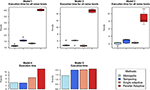Figure 4. Empirical calculation times for 1 million iterations with different MCMC algorithms based on the implementations used in this paper. For models 1–3 boxplots depict the distribution due to repeats with different simulated noise levels. Time execution for models 4 and 5 is represented with barplots. Models were run on a 24 core Intel Xeon E5-2697 machine with 128 GB RAM.

We next analyzed all algorithms with respect to convergence via the Geweke test (single chain MCMC) and Gelman-Rubin statistic (multi-chain MCMC), respectively (Table 1). Accordingly, convergence could be assessed in all cases for parallel tempering and single adaptive MCMC, but not for multi-chain adaptive MCMC. No systematic dependency between noise level and convergence failure could be observed.

TABLE 1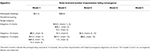Table 1. Cases in which no convergence was observed according to Geweke's (single chains) and Gelman-Rubin's test (parallel tempering).

It is important to highlight here that all convergence tests have their limitations. In particular, the Gelman-Rubin diagnostic may simply fail to identify convergence due to the multi-modality of the posterior distribution . Similarly, the Geweke test tries to verify a necessary but not sufficient condition for convergence. Thus, the test can only provide hints, whether convergence has likely failed, but not when it has been achieved.

#### Influence of Informative Priors on Convergence

Next, we investigated whether there was an influence of informative prior distributions for noise variance (Equation 4) and rate parameters (Equation 5) on convergence. Since informative priors should be of particular use in situations with higher measurement noise, we only performed this comparison for noise levels 5, 10, 15, and 25%. Moreover, we restricted this assessment to Metropolis-Hastings and single chain adaptive MCMC. According to the results shown in Table 2 no clear influence of informative priors on convergence could be identified for Metropolis-Hastings, but in case of single adaptive MCMC all models showed convergence when incorporating an informative prior for rate parameters.

TABLE 2

### Effective Sample Size

#### Parallel MCMC Yields More Effective Sampling

We compared the ESS achieved by the different MCMC algorithms while not using any informative prior and fixing the noise level to 10% (Figure 5). Interestingly, our results indicate the most effective sampling by parallel tempering for models 1, 4, and 5, while for the other two models parallel adaptive MCMC seemed to be most efficient. We speculate that in case of non-trivial correlations among parameters adaptive MCMC might yield a higher ESS. Altogether, parallel MCMC techniques seem to provide an advantage compared to single chain methods, which matches the intuition.

FIGURE 5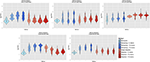Figure 5. Effective sample size achieved by different MCMC algorithms per model. The y-axis is on log-scale.

#### Influence of Informative Priors on Effective Sample Size

We next investigated, whether informative priors would enhance the effective sampling size. We focused this comparison on single chain adaptive MCMC and Metropolis-Hastings with the same noise level as in the previous paragraph. Interestingly, a certain advantage of informative priors could only be observed for adaptive MCMC (Figure 6). However, the exact type of prior that yielded most effective sampling was not identical for all models, and for model 4 no influence of the prior on effective sampling size could be observed.

FIGURE 6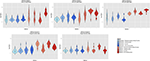Figure 6. Influence of informative Bayesian priors on effective sample size. The y-axis is on log-scale.

### Parameter Estimates

We compared the fraction of true model parameters lying within the 95% Bayesian credible interval of the posterior distribution. Our results indicate a clear benefit for parallel adaptive MCMC for all models and noise levels (Figure 7). Interestingly, parallel adaptive MCMC seems highly robust against noise, because the fraction of true parameters lying within the 95% Bayesian credible interval was not obviously affected. Also on experimental data parallel adaptive MCMC resulted into a high fraction of true parameters within the 95% Bayesian credible interval, even with only 5 parallel chains. Parallel tempering showed a quite unsystematic dependency on noise level, and altogether the fraction of true model parameters covered by the credible interval was much lower than for parallel adaptive MCMC. This indicates that learning the covariance structure of model parameters, as done by parallel adaptive MCMC methods, is probably primarily beneficial for an effective exploration of different modes of the posterior distribution. Different parallel chains begin to explore different regions of the posterior, and the covariance structure seems to guide the focus on those regions.

FIGURE 7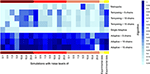Figure 7. Heatmap for the fraction of true parameters lying into the 95% Bayesian credible interval of MCMC samples. Samples were estimated by single and multiple parallel MCMC. X-axis is labeled with noise levels used in the fiver models (dark red color bar, model 1; red, model 2; light red, model 3; light yellow, model 4; yellow, model 5).

We explored whether our suggested informative priors could improve parameter estimation, while focusing on single chain MCMC methods. Figure 8 clearly shows that this was the case, also with experimental data. Specifically, a combination of both informative priors yielded a high fraction of true model parameters covered by a Bayesian 95% credible interval. The benefit was most pronounced for adaptive MCMC, and the combined effect of both priors was in models 1–3 stronger than each individual one. In model 5 the informative prior for model parameters in adaptive MCMC yielded the strongest benefit.

FIGURE 8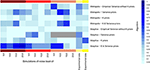Figure 8. Heatmap for the fraction of true parameters lying into the 95% confidence interval of MCMC samples. Samples were estimated through different likelihood computation. X-axis is labeled with noise levels used in the five models, starting with model 1 and ending with models 4 (light yellow) and 5 (dark yellow).

## Conclusion

ODE systems play an important role to describe biological mechanisms in systems biology and systems medicine. However, kinetic rate parameters appearing in these models are typically unknown and have to be inferred from experimental data, which is a challenging problem due to practical and structural non-identifiability of parameters . Full Bayesian inference can in principle address both aspects, but usually requires computationally costly sampling via MCMC. The design of a good sampling algorithm providing sufficiently fast convergence to the target distribution, high ESS, and high probability of coverage of the true parameter is therefore very important. However, this is non-trivial from a statistical point of view. The first aim of this work was to benchmark different MCMC strategies, specifically including parallel MCMC methods that can—at least in theory—deal with the existence of multiple statistical modes of posterior distributions. The second aim was to understand, whether our suggested prior distributions for noise variance and rate parameters were beneficial.

Using five published ODE models we performed extensive comparisons of our tested MCMC algorithms by investigating convergence behavior, ESS, and the quality of parameter estimates. Our results showed that with parallel adaptive MCMC the highest fraction of true model parameters was covered by the 95% Bayesian credible interval of the posterior distribution. In addition, adaptive MCMC methods appeared to benefit most from the inclusion of our suggested informative priors, including convergence. No benefit in terms of convergence could be found for parallel adaptive MCMC compared to other MCMC methods. ESS in general increased with parallel sampling methods (matching the intuition), but this benefit was independent of the type of parallel MCMC approach. Of course, we cannot fully exclude that beyond the impact of the actual MCMC sampling technique there is also some (minor) influence of the actual code implementation used in this paper.

MCMC techniques are per se not without limitations. As mentioned above they are computationally costly due to the large number of required likelihood function evaluations, and with significantly larger ODE systems than the ones used in this work convergence might become more challenging. Notably, some authors have suggested specific approximations to deal with computationally expensive posterior distributions, e.g., via Gaussian Process regression  and local approximations , which might be a principle way to scale the methods investigated in this paper to larger ODE systems.

In summary, according to our results parallel adaptive MCMC in conjunction with the suggested variance prior and prior for kinetic rate parameters seems a promising approach for full Bayesian inference of parameters in ODE based models in systems biology.

## Data Availability Statement

The synthetic datasets for this study were generated from models found in the BioModels Database of the European Bioinformatics Institute (https://www.ebi.ac.uk/biomodels/). Experimental data used in this study can be found in Benchmark Problem Collection Repository (https://doi.org/10.1093/bioinformatics/btz020).

## Author Contributions

GV-B and HF jointly drafted the manuscript. HF defined the research question and supervised the work. All authors read and approved the final manuscript.

## Funding

GV-B was funded by the Panamanian and the German government, through the Secretaría Nacional de Ciencia, Tecnología e Innovación (SENACYT) and the Deutscher Akademischer Austauschdienst (DAAD). Grants numbers for the DAAD-SENACYT scholarship are A/10/90709 (DAAD) and BEA-2010-017 (SENACYT).

## Conflict of Interest

The authors declare that the research was conducted in the absence of any commercial or financial relationships that could be construed as a potential conflict of interest.

## Acknowledgments

We thank the Jülich Supercomputing Center (Dr. Olav Zimmerman) for partially supporting this work by granting access to their computational resources.

## Supplementary Material

The Supplementary Material for this article can be found online at: https://www.frontiersin.org/articles/10.3389/fams.2019.00055/full#supplementary-material

## References

1. Tsimring LS. Noise in biology. Rep Prog Phys. (2014) 77:026601. doi: 10.1088/0034-4885/77/2/026601

2. Hoops S, Sahle S, Gauges R, Lee C, Pahle J, Simus N, et al. COPASI—a COmplex PAthway SImulator. Bioinformatics. (2006) 22:3067–74. doi: 10.1093/bioinformatics/btl485

3. Maiwald T, Timmer J. Dynamical modeling and multi-experiment fitting with PottersWheel. Bioinformatics. (2008) 24:2037–43. doi: 10.1093/bioinformatics/btn350

4. Raue A, Kreutz CMT, Klingmüller U, Timmer J. Addressing parameter identifiability by model-based experimentation. IET Syst Biol. (2011) 5:120–30. doi: 10.1049/iet-syb.2010.0061

5. Raue A, Karlsson J, Saccomani MP, Jirstrand M, Timmer J. Comparison of approaches for parameter identifiability analysis of biological systems. Bioinformatics. (2014) 30:1440–8. doi: 10.1093/bioinformatics/btu006

6. Chis OT, Banga JR, Balsa-Canto E. Structural identifiability of systems biology models: a critical comparison of methods. PLoS ONE. (2011) 6:e27755. doi: 10.1371/journal.pone.0027755

7. Zhan C, Situ W, Yeung LF, Tsang PWM, Yang G. A parameter estimation method for biological systems modelled by ODE/DDE models using spline approximation and differential evolution algorithm. IEEE/ACM Trans Comput Biol Bioinform. (2014) 11:1066–76. doi: 10.1109/TCBB.2014.2322360

8. Peng H, Guo Z, Deng C, Wu Z. Enhancing differential evolution with random neighbors based strategy. J Comput Sci. (2018) 26:501–11. doi: 10.1016/j.jocs.2017.07.010

9. Mathews JD, McCaw CT, McVernon J, McBryde ES, McCaw JM. A biological model for influenza transmission: pandemic planning implications of asymptomatic infection and immunity. PLoS ONE. (2007) 2:e1220. doi: 10.1371/journal.pone.0001220

10. Hamon J, Jennings P, Bois FY. Systems biology modeling of omics data: effect of cyclosporine a on the Nrf2 pathway in human renal cells. BMC Syst Biol. (2014) 8:76. doi: 10.1186/1752-0509-8-76

11. Keersmaekers N, Ogunjimi B, Van Damme P, Beutels P, Hens N. An ODE-based mixed modelling approach for B-and T-cell dynamics induced by Varicella-Zoster Virus vaccines in adults shows higher T-cell proliferation with Shingrix compared to Varilrix. Vaccine. (2019) 37:2537–53. doi: 10.1016/j.vaccine.2019.03.075

12. Metropolis N, Rosenbluth AW, Rosenbluth MN, Teller AH, Teller E. Equation of state calculations by fast computing machines. J Chem Phys. (1953) 21:1087–92. doi: 10.1063/1.1699114

13. Hastings WK. Monte Carlo sampling methods using Markov chains and their applications. Biometrika. (1970) 57:97–109. doi: 10.1093/biomet/57.1.97

14. Haario H, Saksman E, Tamminen J. Adaptive proposal distribution for random walk Metropolis algorithm. Comput Stat. (1999) 14:375–96. doi: 10.1007/s001800050022

15. Geyer CJ, Thompson EA. Annealing Markov chain Monte Carlo with applications to ancestral inference. J Am Stat Assoc. (1995) 90:909–20. doi: 10.1080/01621459.1995.10476590

16. Craiu RV, Rosenthal J, Yang C. Learn from thy neighbor: parallel-chain and regional adaptive MCMC. J Am Stat Assoc. (2009) 104:1454–66. doi: 10.1198/jasa.2009.tm08393

17. Vihola M. Robust adaptive Metropolis algorithm with coerced acceptance rate. Stat Comput. (2012) 22:997–1008. doi: 10.1007/s11222-011-9269-5

18. Ballnus B, Hug S, Hatz K, Görlitz L, Hasenauer J, Theis FJ. Comprehensive benchmarking of Markov chain Monte Carlo methods for dynamical systems. BMC Syst Biol. (2017) 11:63. doi: 10.1186/s12918-017-0433-1

19. Chelliah V, Juty N, Ajmera I, Ali R, Dumousseau M, Glont M, et al. BioModels: ten-year anniversary. Nucleic Acids Res. (2014) 43:D542–8. doi: 10.1093/nar/gku1181

20. Kholodenko BN. Negative feedback and ultrasensitivity can bring about oscillations in the mitogen-activated protein kinase cascades. Eur J Biochem. (2000) 267:1583–8. doi: 10.1046/j.1432-1327.2000.01197.x

21. Schilling M, Maiwald T, Hengl S, Winter D, Kreutz C, Kolch W, et al. Theoretical and experimental analysis links isoform-specific ERK signalling to cell fate decisions. Mol Syst Biol. (2009) 5:334. doi: 10.1038/msb.2009.91

22. Clarke DC, Betterton M, Liu X. Systems theory of Smad signalling. Syst Biol. (2006) 153:412–24. doi: 10.1049/ip-syb:20050055

23. Brännmark C, Palmer R, Glad ST, Cedersund G, Stralfors P. Mass and information feedbacks through receptor endocytosis govern insulin signaling as revealed using a parameter-free modeling framewor. J Biol Chem. (2010) 285:20171–9. doi: 10.1074/jbc.M110.106849

24. Hass H, Loos C, Alvarez ER, Timmer J, Hasenauer J, Kreutz C. Benchmark problems for dynamic modeling of intracellular processes. Bioinformatics. (2019) 35:3073–82. doi: 10.1101/404590

25. Raia V, Schilling M, Böhm M, Hahn B, Kowarsch A, Raue A, et al. Dynamic mathematical modeling of IL13-induced signaling in Hodgkin and primary mediastinal B-cell lymphoma allows prediction of therapeutic targets. Cancer Res. (2011) 71:693–704. doi: 10.1158/0008-5472.CAN-10-2987

26. Zhu C. Algorithm 778: L-BFGS-B: Fortran subroutines for large-scale bound-constrained optimization. J ACM Trans Math Softw. (1997) 23:550–60. doi: 10.1145/279232.279236

27. Hindmarsh AC, Sherman AH. LSODES: Livermore Solver for Ordinary Differential Equations With General Sparse Jacobian Matrices. Livermore, CA: Lawrence Livermore National Laboratory (1982).

28. Zacher B, Abnaof K, Gade S, Younesi E, Tresch A, Fröhlich H. Joint Bayesian inference of condition-specific miRNA and transcription factor activities from combined gene and microRNA expression data. Bioinformatics. (2012) 28:1714–20. doi: 10.1093/bioinformatics/bts257

29. Schomburg I, Chang A, Hofmann O, Ebeling C, Ehrentreich F, Schomburg D. BRENDA: a resource for enzyme data and metabolic information. Trends Biochem Sci. (2002) 27:54–6. doi: 10.1016/S0968-0004(01)02027-8

30. Jeske L, Placzek S, Schomburg I, Chang A, Schomburg D. BRENDA in 2019: a European ELIXIR core data resource. Nucleic Acids Res. (2018) 47:D542–9. doi: 10.1093/nar/gky1048

31. Scheidegger A. adaptMCMC: Implementation of a Generic Adaptive Monte Carlo Markov Chain Sampler. (2012).

32. Geweke J. Evaluating the Accuracy of Sampling-Based Approaches to the Calculation of Posterior Moments. Minneapolis, MN: Federal Reserve Bank of Minneapolis, Research Department Minneapolis, MN (1991).

33. Holm S. A simple sequentially rejective multiple test procedure. Scand J Stat. (1979) 6:65–70.

34. Gelman A, Rubin DB. Inference from iterative simulation using multiple sequences. Stat Sci. (1992) 7:457–72. doi: 10.1214/ss/1177011136

35. Plummer M, Best N, Cowles K, Vines K. CODA: Convergence Diagnosis and Output Analysis for MCMC. R News. (2006) 6:7–11. Available online at: https://cran.r-project.org/doc/Rnews/Rnews_2006-1.pdf#page=7

36. Baker CT, Bocharov G, Ford JM, Lumb PM, Norton SJ, Paul C, et al. Computational approaches to parameter estimation and model selection in immunology. J Comput Appl Math. (2005) 184:50–76. doi: 10.1016/j.cam.2005.02.003

37. Kreutz C, Raue A, Kaschek D, Timmer J. Profile likelihood in systems biology. FEBS J. (2013) 280:2564–71. doi: 10.1111/febs.12276

38. Ashyraliyev M, Fomekong-Nanfack Y, Kaandorp JA, Blom JG. Systems biology: parameter estimation for biochemical models. FEBS J. (2009) 276:886–902. doi: 10.1111/j.1742-4658.2008.06844.x

39. Brooks SP, Roberts GO. Assessing convergence of Markov chain Monte Carlo algorithms. Stat Comput. (1998) 8:319–35.

40. Fielding M, Nott DJ, Liong SY. Efficient MCMC schemes for computationally expensive posterior distributions. Technometrics. (2011) 53:16–28. doi: 10.1198/TECH.2010.09195

41. Conrad PR, Marzouk YM, Pillai NS, Smith A. Accelerating asymptotically exact MCMC for computationally intensive models via local approximations. J Am Stat Assoc. (2016) 111:1591–607. doi: 10.1080/01621459.2015.1096787

Keywords: Bayesian inference, parameter estimation, ODE models, Metropolis-Hastings, adaptive MCMC, parallel tempering MCMC, likelihood computation

Citation: Valderrama-Bahamóndez GI and Fröhlich H (2019) MCMC Techniques for Parameter Estimation of ODE Based Models in Systems Biology. Front. Appl. Math. Stat. 5:55. doi: 10.3389/fams.2019.00055

Received: 18 May 2019; Accepted: 17 October 2019;
Published: 01 November 2019.

Edited by:

Matteo Barberis, University of Surrey, United Kingdom

Reviewed by:

Fabian Froehlich, Harvard Medical School, United States
Monika Heiner, Brandenburg University of Technology Cottbus-Senftenberg, Germany

Copyright © 2019 Valderrama-Bahamóndez and Fröhlich. This is an open-access article distributed under the terms of the Creative Commons Attribution License (CC BY). The use, distribution or reproduction in other forums is permitted, provided the original author(s) and the copyright owner(s) are credited and that the original publication in this journal is cited, in accordance with accepted academic practice. No use, distribution or reproduction is permitted which does not comply with these terms.

*Correspondence: Gloria I. Valderrama-Bahamóndez, bahamond@bit.uni-bonn.de; gloria.valderrama@utp.ac.pa